Precalculus : Find the Area of a Segment of a Circle

Example Questions

Example Question #1 : Find The Area Of A Segment Of A Circle

On a Cartesian plane plot a circle centered at the origin of radius. Now draw a segment from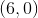to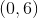. Shade the area between the segment and the boundary of the circle, above the segment.

What is the area of the shaded region?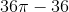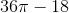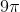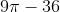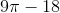Explanation:

The shaded area is one quarter of the area of the circle, minus the area of an isosceles right triangle with legs along the radius.

The circle has radius, so the area of the circle is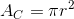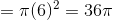The part of the circle in the first quadrant is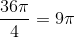If we subtract the area under the line connecting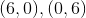we get the correct answer.

To find this area, recognize the geometry as a triangle. The two points give you the base and height so its area is,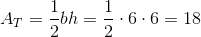Therefore, the area of the region of interest is.

Example Question #2 : Find The Area Of A Segment Of A Circle

Find the area of the shaded segment of the given circle that has a radius of 8 cm: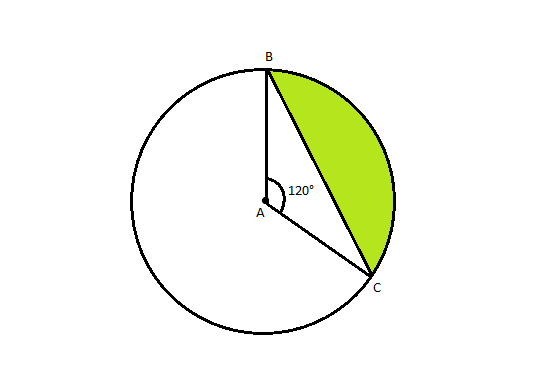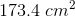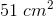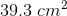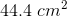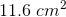Explanation:

The area of a segment can be calculated with the following formula: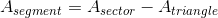Finding the area of the sector is calculated as such: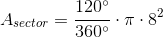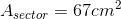To find the area of the triangle, the 120 degree isosceles can be divided into two 30 60 90 triangles: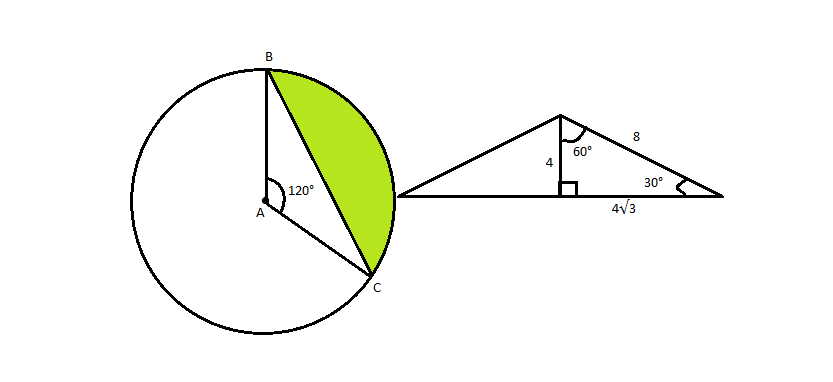The area for the triangle can be calculated as such: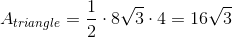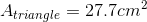Going back to the original formula for segment area: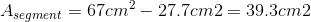Example Question #3 : Find The Area Of A Segment Of A Circle

Find the area of the shaded segment: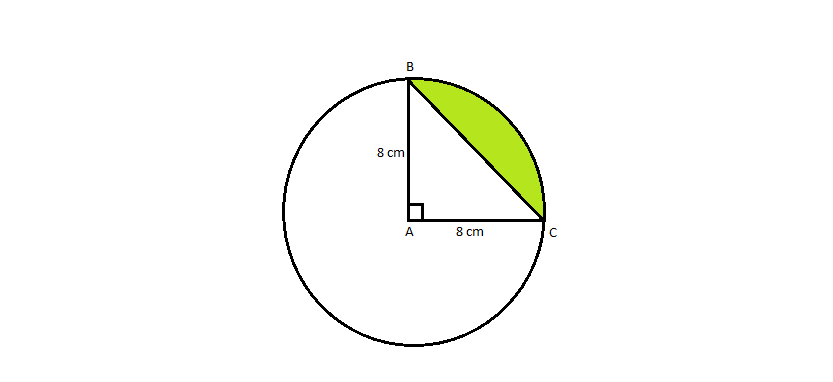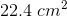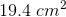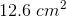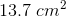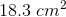Explanation:

The area of the segment can be calculated with the following equation:The area of the sector can be found as such: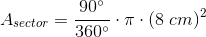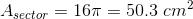The area of the triangle can be determined easily because it is a right triangle, at 90 degrees: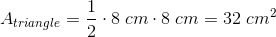Now that we have both variables needed for the formula, we can determine the area of the segment: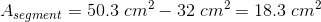Example Question #4 : Find The Area Of A Segment Of A Circle

Find the area of the shaded segment BC: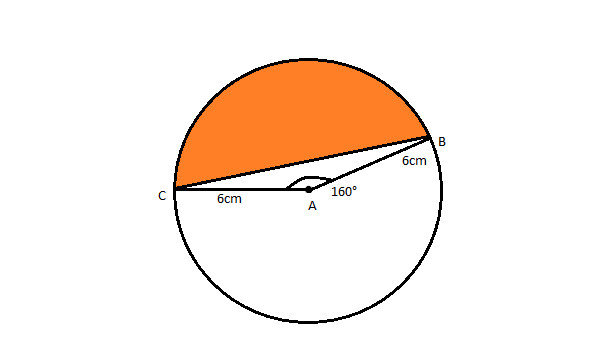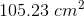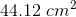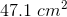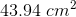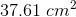Explanation:

The area of a segment can be calculated as such:We can find the area of the sector as such: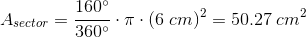To find the area of the triangle, we must divide the isosceles into two right triangles with a bisector: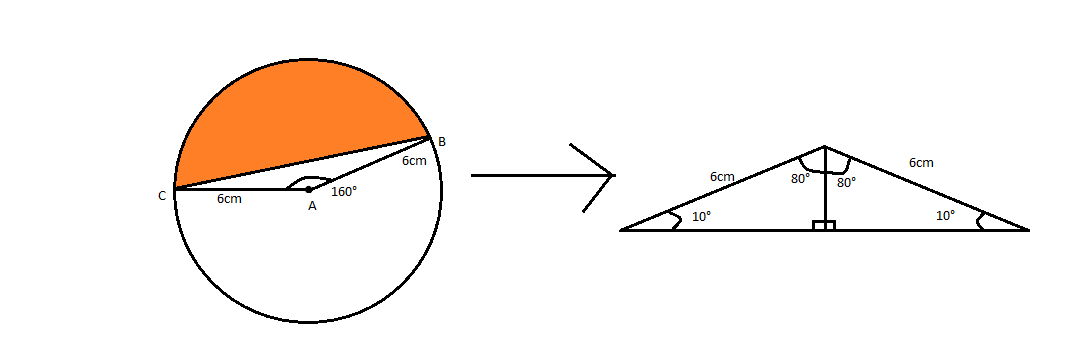To determine base, we calculate: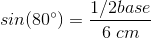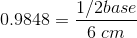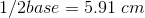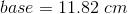To determine height, we calculate: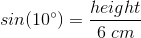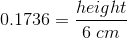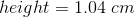Now that we have all the needed values, we can calculate the area of the triangle: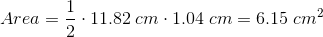The total segment area for BC can now be calculated: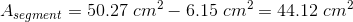Example Question #5 : Find The Area Of A Segment Of A Circle

Find the area of the segment BC: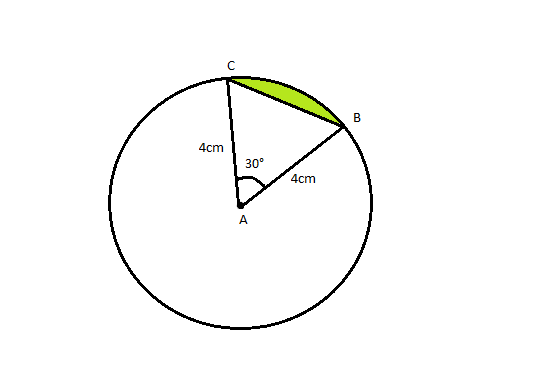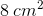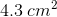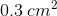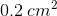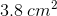Explanation:

To solve for the area of the segment, we must first find the area of the sector and the triangle, to fulfill the given formula:To find the area of the sector: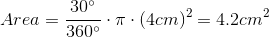To find the area of the triangle, we first must divide it into two right triangles: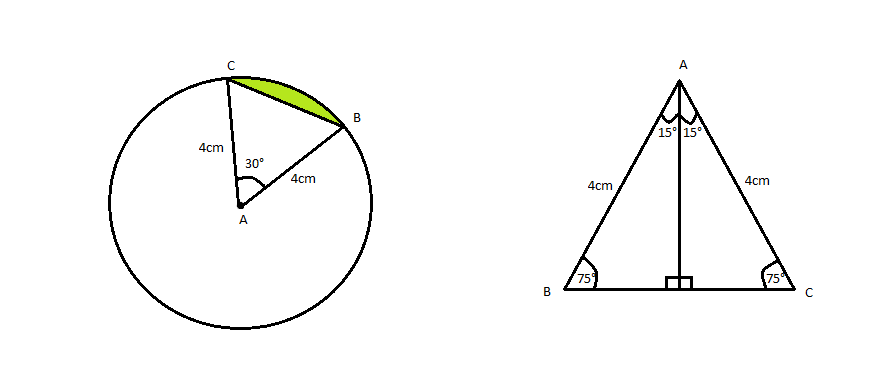We can find the height of the triangle as such: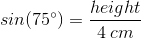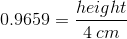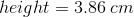We can find the base employing a similar method: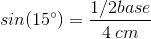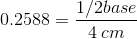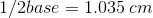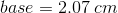The area of the triangle can then be calculated as such: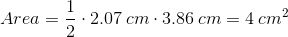To find the Area of the segment, we can now subtract the triangle area from the sector area: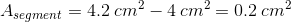Example Question #6 : Find The Area Of A Segment Of A Circle

Find the area of a quarter circle with radius 9cm.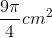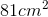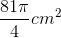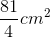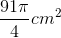To find the area of a quarter circle, first remember what the area of a circle is. The formula is. Since we're only interested in a quarter of the circle, let's use the formula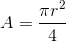. Then, plug in the radius so that your answer is:.